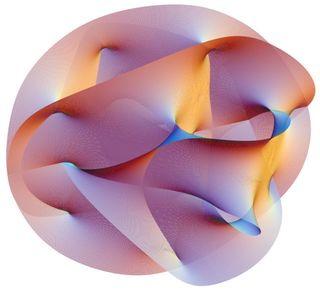# String Theorists Simulate the Big BangJapanese physicists have created a string theory model that simulates the birth of the universe. In their model, the Big Bang was a "symmetry-breaking event" — a fluctuation that caused three spatial dimensions to break free from the other six dimensions of string theory, then rapidly unfurl to produce our universe's observed 3D structure.

String theory — a proposed "theory of everything" that unites quantum mechanics and general relativity together in one complete picture — models elementary particles as oscillating lines ("strings") rather than dimensionless points. In order for the math to work, string theory requires that there be 10 dimensions: nine of space and one of time. Our universe only appears to have three spatial dimensions, string theorists say, because the other six are curled up in undetectably tiny bundles called Calabi-Yau manifolds, which are a minuscule 10^-33 centimeters across.

Like many other underlying aspects of string theory, there's no feasible experiment that could verify that these manifolds exist, and thus that the universe really does have a 9-dimensional spatial structure. And if it does, physicists wonder why three of those dimensions would be enormous and the other six extremely small. Nonetheless, the string theory framework is compelling because it gracefully explains most aspects of the universe that we do observe, from electromagnetism to gravity to the thermodynamics of black holes.

The new research — by Sang-Woo Kim of Osaka University, Jun Nishimura of the High Energy Accelerator Research Organization (KEK) and Asato Tsuchiya of Shizuoka University — shows that string theory plausibly accounts for the universe's origin and its apparent 3D structure. "We have been able to see how three directions start to expand at some point in time," Nishimura told Life's Little Mysteries. [Does the Universe Have an Edge?]

To do the calculation, the physicists used a reformulation of string theory called the IIB matrix model, which couches the theory in the form of matrices — rectangular grids of mathematical expressions that describe the properties of the universe. The elements of the matrices can be mathematically combined to simulate how strings might have interacted to form the universe as it's seen today.

"In the matrix model formulation for superstring theory, matrices are supposed to be the fundamental degrees of freedom, and the string picture emerges when one takes the limit of infinitely large matrix size," Nishimura wrote in an email. Infinitely large matrices being unwieldy, the physicists approximated the structure of the universe by working with finite matrices, the largest of which had 32 rows and 32 columns.

The usual method of doing string theory calculations is to use perturbation theory, in which all possible interactions between strings are considered together in a calculation of a single interaction. But the equations describing string interactions are so complicated that this method only works when all the possible interactions between strings are very weak — small perturbations rather than the high-energy collisions and compactifications that would have happened in the early universe. Matrices, on the other hand, can be numerically simulated by supercomputers, and so the reformulation of string theory into a matrix model is enabling physicists to calculate how events would unfold at extremely high energies, such as those at the moment of the Big Bang. "This was not possible with the conventional formulation of superstring theory," Nishimura wrote.

"What we do in this simulation is to generate hundreds or thousands of matrices, each of which describes the whole history of the universe during some finite time interval. We then have to take an average over the matrices to get the physical information as to how the universe evolves in time," Nishimura explained.

Larger matrices offered glimpses of larger windows in time. Simulations using 32 x 32 matrices, the largest in the experiment, took two months to complete on a supercomputer, and allowed the researchers to watch the evolution of the universe from the Big Bang until the onset of inflation — a period of exponential expansion — just 10^-36 seconds later.

A key result of the experiment was that, all by itself, the nine-dimensional model universe spontaneously ballooned in three directions, while its six other spatial dimensions remained tightly wrapped. This symmetry-breaking event was described by the changing rows and columns of variables in the matrices; mathematical operations on the matrices produced the coordinates of space, and with each time step, the coordinates increased in three directions (while remaining unchanged in the other six). To those who could interpret them, the changing matrices expressed, in mathematical terms, the birth of space-time.

The researchers said the spontaneous symmetry-breaking resulted from a quantum fluctuation — a momentary violation in the law of conservation of energy, which is permitted by the rules of quantum mechanics. "The space-time has certain uncertainties … as dictated by Heisenberg's uncertainty relation. One of our important findings is that this quantum nature of space-time indeed favors three extended spatial directions rather than other cases. How the quantum space-time evolves into a classical one as we perceive now, is an important issue we are planning to address in the near future," Nishimura wrote.

To investigate this and other questions about the evolution of the universe according to string theory, the researchers must probe a window of time beyond the start of inflation, and to do this, they must simulate even larger matrices. "We are working on it now," he noted.

The new research will be detailed in a forthcoming paper in the journal Physical Review Letters.